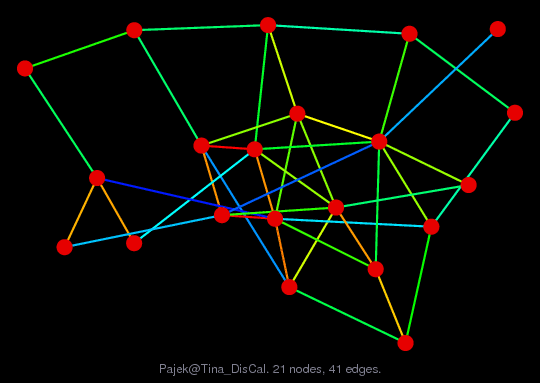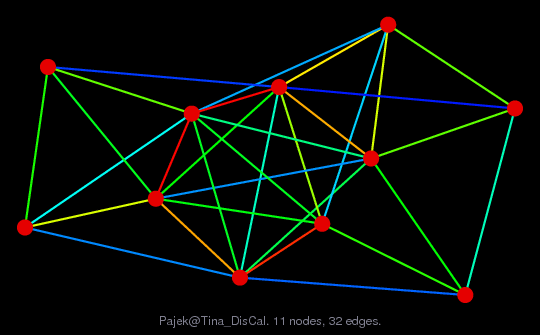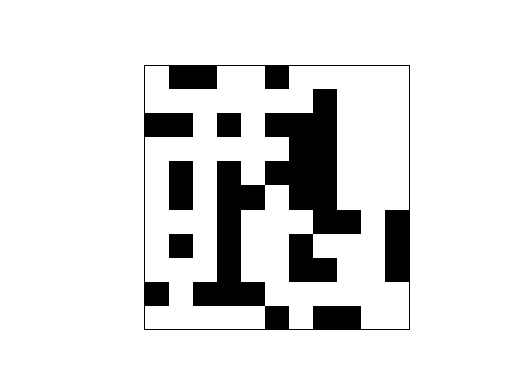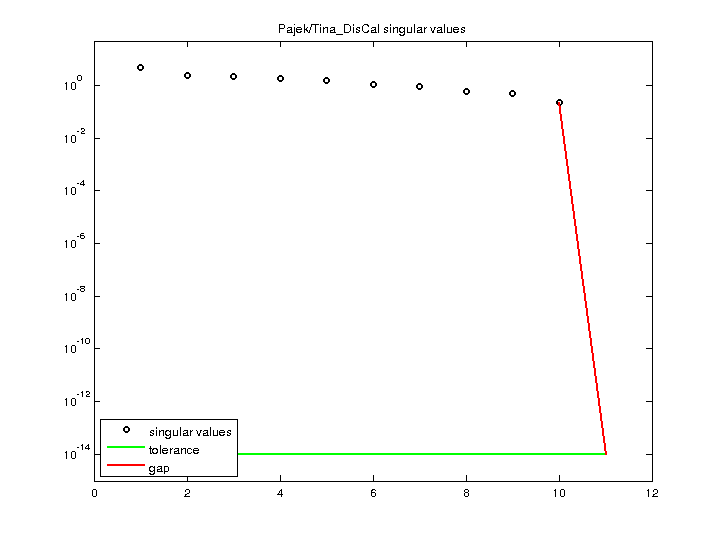Matrix: Pajek/Tina_DisCal

Description: Pajek network: student govt, Univ. Ljubljana, 1992 (discuss, recall)(bipartite graph drawing) (graph drawing of A+A')• Matrix group: Pajek
• download as a MATLAB mat-file, file size: 1 KB. Use UFget(1527) or UFget('Pajek/Tina_DisCal') in MATLAB.

 Matrix properties number of rows 11 number of columns 11 nonzeros 41 # strongly connected comp. 3 explicit zero entries 0 nonzero pattern symmetry 44% numeric value symmetry 44% type binary structure unsymmetric Cholesky candidate? no positive definite? no

 author V. Batagelj editor V. Batagelj date 1992 kind directed graph 2D/3D problem? no

 Additional fields size and type nodename full 11-by-9 coord full 11-by-2

Notes:

```------------------------------------------------------------------------------
Pajek network converted to sparse adjacency matrix for inclusion in UF sparse
matrix collection, Tim Davis.  For Pajek datasets, See V. Batagelj & A. Mrvar,
------------------------------------------------------------------------------
The original problem had 3D xyz coordinates, but all values of z were equal
to 0, and have been removed.  This graph has 2D coordinates.
```

 SVD-based statistics: norm(A) 4.78646 min(svd(A)) 0 cond(A) Inf rank(A) 10 null space dimension 1 full numerical rank? no singular value gap Inf

 singular values (MAT file): click here SVD method used: s = svd (full (A)) status: ok